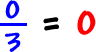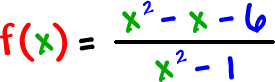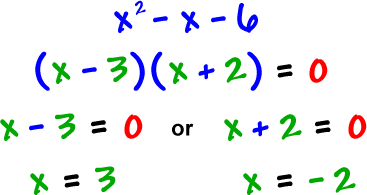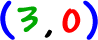X-INTERCEPTS:  Where the graph crosses the x-axis

Hey, this is the same as finding the zeros!

 Setand solve

But, here's the cool thing:  For these rational guys, you just need to set the numerator = 0 and solve!

Well, when a fraction is 0, it's the same as saying the numerator is 0:So, set the numerator = 0 and solve.

Check it out:numerator = 0So, the x-intercepts are the pointsandTRY IT:

Find the x-intercepts ofBIG NOTE:

 These processes find the ONLY intercepts! There will NOT be any others!!

(This info will be very important.)